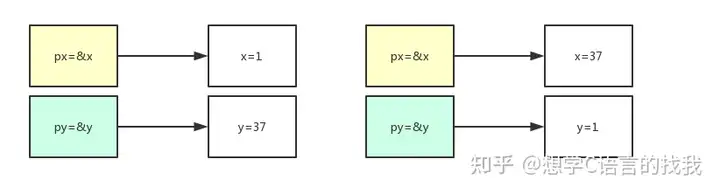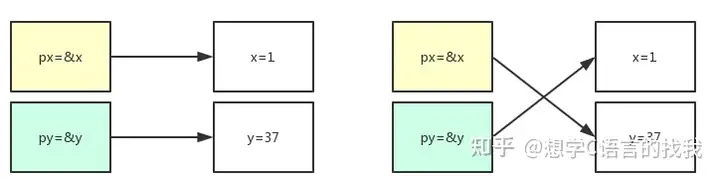# 【C语言】交换函数（swap）

## 【C语言】交换函数（swap）

void swap(int x, int y) {

int temp = y;

y = x;

x = temp;

}

int main()

{

int x = 1;

int y = 37;

swap(x, y);

printf(“x:%d; y:%d\n”,x,y);

return 0;

}

【原因解析】：

void pswap(int *x, int *y) {

int temp = *y;

*y = *x; //将x指针所指地址的值 赋值给 y指针所指地址；*x相当于一个整数，将其赋值给指针变量所指的地址

*x = temp;

}

void prswap(int *&x, int *&y) {

int temp = *y;

*y = *x;

*x = temp;

}

void prswap(int *&x, int *&y) {

int *temp = y;

y = x;

x = temp;

}

（1）交换内存中的值（2）交换指针地址View All Posts